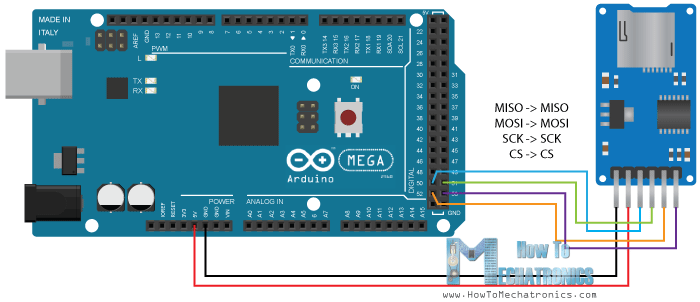Hi everybody! I am a beginner with Arduino. But with this project I am working on right now I have the same problem over and over.

Setup: I got a GY-521 Accelerometer connected to my Arduino.The internet tells me that I only have to use the SCL and SDA wires, so I did. The sensor is green and everything but when I upload the code, every time I check out the Serial Monitor to see the data I get question marks. Like thisAt first It seems to work but then the data turns into question marks. I really don't know how I can fiks this, the only thing I can think of is that maybe my wire.h library is not installed properly or anything.

I've used many different tutorials for example:

When I got this fiksed and got the code well. I want to make a device what works together with an micro SD card reader.Anyone extra tips for that?

This is the code I was using right now:

``````#include
#define Power_Register 0x2D
#define Y_Axis_Register_DATAY0 0x34
#define Y_Axis_Register_DATAY1 0x35
#define Z_Axis_Register_DATAZ0 0x36
#define Z_Axis_Register_DATAZ1 0x37
int X0,X1,X_out;
int Y0,Y1,Y_out;
int Z1,Z0,Z_out;
float Xa,Ya,Za;
void setup() {
Wire.begin(); // Initiate the Wire library
Serial.begin(9600);
delay(100);

Wire.write(Power_Register); // Power_CTL Register
// Enable measurement
Wire.write(8); // Bit D3 High for measuring enable (0000 1000)
Wire.endTransmission();
}
void loop() {
// X-axis
//Ask the particular registers for data
Wire.write(X_Axis_Register_DATAX0);
Wire.write(X_Axis_Register_DATAX1);
Wire.endTransmission(); // Ends the transmission and transmits the data from the two registers
if(Wire.available()<=2) {  //
/* Converting the raw data of the X-Axis into X-Axis Acceleration
- The output data is Two's complement
- X0 as the least significant byte
- X1 as the most significant byte */
X1=X1<<8;
X_out =X0+X1;
Xa=X_out/256.0; // Xa = output value from -1 to +1, Gravity acceleration acting on the X-Axis
}
// Y-Axis
Wire.write(Y_Axis_Register_DATAY0);
Wire.write(Y_Axis_Register_DATAY1);
Wire.endTransmission();
if(Wire.available()<=2) {
Y1=Y1<<8;
Y_out =Y0+Y1;
Ya=Y_out/256.0;
}
// Z-Axis
Wire.write(Z_Axis_Register_DATAZ0);
Wire.write(Z_Axis_Register_DATAZ1);
Wire.endTransmission();
if(Wire.available()<=2) {
Z1=Z1<<8;
Z_out =Z0+Z1;
Za=Z_out/256.0;
}
// Prints the data on the Serial Monitor
Serial.print("Xa= ");
Serial.print(Xa);
Serial.print("   Ya= ");
Serial.print(Ya);
Serial.print("   Za= ");
Serial.println(Za);
}
``````

Please post the code that you are using.

OP’s imageThe internet tells me that I only have to use the SCL and SDA wires

Which is true with only one device selected, but you cannot leave the chip select pin floating.

Need to post you code inside code tags to get rid of the smiling faces ;)